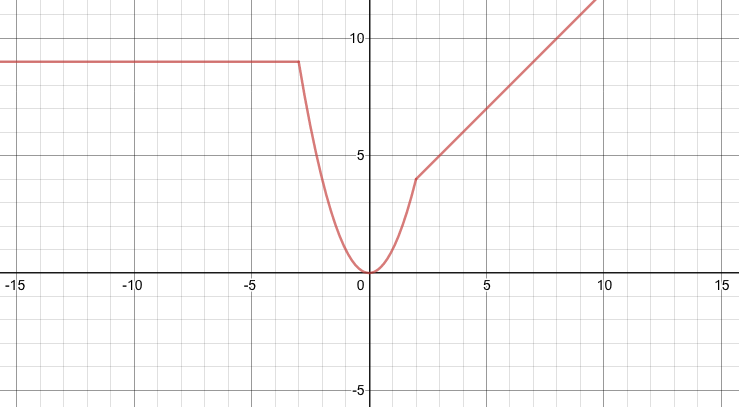# hectictar

 Username hectictar Score 8956 StatsQuestions 10 Answers 2843

+2
30
2
hectictar  May 29, 2020, 10:02 PM
+4
1
1064
3

hectictar  Sep 9, 2018
+4
1
1066
2

### Decomposing a Vector

hectictar  Apr 25, 2018
+11
1
1204
4

### Melody's Birthday!!!

off-topic
hectictar  Apr 15, 2018
+2
1
1607
3
hectictar  Sep 20, 2017
+1
1
1561
2

hectictar  Apr 25, 2017
+1
1
1559
4
hectictar  Mar 29, 2017
#8
+3

Examples:

The greatest common divisor of  6  and  9

=   gcd(6, 9)   ←The difference between 6 and 9 is  3, so replace the larger number, 9, with 3.

=   gcd(6, 3)   ←The difference between 6 and 3 is  3, so replace the larger number, 6, with 3.

=   gcd(3, 3)   ←Now the two numbers are the same. So we stop.

=   3

The greatest common divisor of  8  and  12

=   gcd(8, 12)

=   gcd(8, 4)

=   gcd(4, 4)

=   4

The greatest common divisor of  24  and  81

=   gcd(24, 81)

=   gcd(24, 57)

=   gcd(24, 33)

=   gcd(24, 9)

=   gcd(15, 9)

=   gcd(6, 9)

=   gcd(6, 3)

=   gcd(3, 3)

=   3

To calculate the greatest common divisor of  3  different numbers, we can use this prinicple:

gcd(a, b, c)   =   gcd( a, gcd(b, c) )

So we apply the Euclidean algorithm twice. Let's see if this works!

gcd(9118, 12173, 33182)   =   gcd( 9118, gcd(12173, 33182) )

First, use the Euclidean algorithm to find the inner piece.

=   gcd(12173, 33182)

=   gcd(12173, 21009)

=   gcd(12173, 8836)

=   gcd(3337, 8836)

=   gcd(3337, 5499)

=   gcd(3337, 2162)

=   gcd(1175, 2162)

=   gcd(1175, 987)

=   gcd(188, 987)

=   gcd(188, 799)

=   gcd(188, 611)

=   gcd(188, 423)

=   gcd(188, 235)

=   gcd(188, 47)

=   gcd(141, 47)

=   gcd(94, 47)

=   gcd(47, 47)

=   47

Next, use the Euclidean algorithm to find the outer piece.

gcd( 9118, gcd(12173, 33182) )

=   gcd(9118, 47)

=   gcd(9071, 47)

=   gcd(9024, 47)

.

.   *Note that   9118 - 47*193  =  47   so we would have to subtract  47  a total of  193  times.*

.

=   gcd(141, 47)

=   gcd(94, 47)

=   gcd(47, 47)

=   47

Nov 13, 2019
#1
+3

We put one open curly brace beside the "pieces" of the piecewise function, for example:

$$f(x)=\begin{cases} x+2 & \text{if}&x> 2 \\ x^2 &\text{if}& -3< x\leq 2 \\ 9 &\text{if}& x\leq-3 \end{cases}$$

This is the way to show the pieces of a piecewise function.

On the other hand, domains and ranges (and, of course, intervals) can be expressed in interval notation.

An interval is a set of values or numbers - not a set of expressions or functions.

E.g., the interval  [0, 5)  is every number between  0  and  5, including 0 and excluding 5.

And by looking at the graph for this function:We can see:

 The domain is: _ (-∞, ∞) The range is: [0, ∞) The intervals of decrease are: (-3, 0) ← This means the function is decreasing for all x values in the interval  (-3, 0) For instance, when x = -1, the function is decreasing. The intervals of increase are: (0, 2)   and   (2, ∞)

All of those are intervals expressed in interval notation.

(At the points where  x = 2  and  x = -3 ,  the slope is undefined, and so the function is neither increasing nor decreasing, and so we do not include those values in the intervals of increase or decrease.)

If the graph passes the vertical line test, then it is a function.

Here are a bunch of examples: https://imgur.com/a/Px4lYIt

(If you still are wondering about whether a particular situation is considered a function or not, you could try to draw it on desmos and share the graph or draw it on a piece of paper and take a photo.)

Hope this helps somewhat! If you're confused about something, please say so!Oct 29, 2019
#1
+2

f(x)  =  x h(x)  =  13x + 1

By the transitive property of equality,

f(x)  =  13x + 1

x h(x)  =  13x + 1     Divide both sides of this equation by  x

h(x)  =  13 + 1/x

Here is the graph of  h:  https://www.desmos.com/calculator/bbndyocwqm

*edit* Now I realize this answer is probably totally wrong, but I will leave it anyway...LOL

Oct 28, 2019